﻿ New Multiplier/Divider Using a Single Cdba

### New Multiplier/Divider Using a Single Cdba

J. K. Pathak, A. K. Singh, Raj SenaniOPEN ACCESSPEER-REVIEWED

## New Multiplier/Divider Using a Single Cdba

J. K. Pathak1, A. K. Singh2, Raj Senani3,1Department of Electronics and Communication Engineering, Echelon Institute of Technology, Faridabad, India

2Departments of Electronics and Communication Engineering, Faculty of Engineering and Technology, HRCT Group of Institutions, Morta, Ghaziabad, India

3Division of Electronics and Communication Engineering, Netaji Subhas Institute of Technology, Sector 3, Dwarka, New Delhi, India

### Abstract

A new multiplier-divider circuit using a single Current Differencing Buffered Amplifier (CDBA) and only six MOSFETs has been presented. The proposed circuit has the advantage of simultaneously realizing a multiplier and divider without changing the circuit topology. The basic functions of the proposed circuit have been verified through PSPICE simulations using a CMOS CDBA and NMOS transistors with process parameters of 0.35 µm CMOS technology and some application results of the proposed cell in various modes of operation have been included.

### At a glance: Figures

123
Prev Next

• Pathak, J. K., A. K. Singh, and Raj Senani. "New Multiplier/Divider Using a Single Cdba." American Journal of Electrical and Electronic Engineering 2.3 (2014): 98-102.
• Pathak, J. K. , Singh, A. K. , & Senani, R. (2014). New Multiplier/Divider Using a Single Cdba. American Journal of Electrical and Electronic Engineering, 2(3), 98-102.
• Pathak, J. K., A. K. Singh, and Raj Senani. "New Multiplier/Divider Using a Single Cdba." American Journal of Electrical and Electronic Engineering 2, no. 3 (2014): 98-102.

 Import into BibTeX Import into EndNote Import into RefMan Import into RefWorks

### 1. Introduction

The Current differencing buffered amplifier (CDBA)  and its current-controlled version have received significant attention in literature as they have been shown to offer a lot of flexibility and versatility in analog circuit design, for instance, see [2, 3, 4, 15, 16, 17, 18] and the references cited therein. Several bipolar and CMOS implementations of the CDBA have also been advanced over the years such as those in [1, 2, 3, 4] and [16-22].

The circuit symbol of the CDBA is shown in Figure 1. A CDBA is a four terminal building block which is characterized by the terminal equations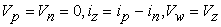(1)

Various researchers have proposed analog multiplier and/or divider circuits in the past using a variety of active building blocks such as Current Controlled Current Conveyors (CCCII) [5, 7], second generation Current Conveyors (CCII) , operational trans-conductance amplifiers (OTA) , current differencing trans conductance amplifiers (CDTA) , operational amplifiers [10, 11, 12, 19], operational trans-resistance amplifier (OTRA) , current feedback operational amplifiers (CFOA) , current controllable current conveyor trans conductance amplifier (CC-CCTA) , dual-X second-generation current conveyors (DXCCIIs)  and current controlled current-differencing trans-conductance amplifiers (CC-CDTA) .

These multiplier/divider circuits, however, suffer from one or other problems. For example, the four quadrant multiplier and two quadrant divider reported in  has the disadvantage of employing two active elements (CCCII+s) elements are the performance of the circuit is temperature-sensitive. Similarly, the four quadrant analog multiplier presented in  also needs two active elements (CCIIs). On the other hand, the current mode (CM) multiplier proposed in  based on bipolar CCCII+ uses one active element and a current controlled ground resistor, requires current-matching for successful operation as multiplier, is temperature-sensitive, has non-differential current inputs and suffers from the effects of the parasitic impedances of the CCCII+. The OTA-based CM analog multiplier-divider reported in  uses too many active elements (three OTAs). The CM analog multiplier and divider introduced in  use two CC-CDTAs but have non-differential inputs. The circuits of [10, 11] realize a multiplier/divider using a single op-amp and eight MOSFETs but the circuit employs both positive and negative feedback thereby leading to a condition for the stability while that of  although reduces the number of MOSFETs to six but needs two op-amps to realize multiplier/divider. Furthermore, as these circuits [10, 11, 12] are based on op-amps, their operation is limited by the well-known limitations of the op-amps. Using a single OTRA and eight MOS transistors, an analog multiplier was reported in  but this circuit has the limitation of allowing only non-differential inputs. In , Liu reported a circuit using only four MOSFETs and two CFOAs{1}, but the circuit therein realizes only a divider. In , Riewruja and Rerkratn reported a four-quadrant multiplier using op-amps but the circuit requires as many as five op-amps and eleven resistors and is hence, not economical. Woratraijariya, Mano, Jaikla, and Maneewan in  reported a CM four quadrant divider, using a single CC-CCTA but the circuit can perform only the operation of a divider. Kumngern  presented a four-quadrant multiplier based on two DXCCIIs but the circuit can perform only multiplication. Siripruchyanun and Jaikla  introduced a CM analog multiplier/divider based on CC-CDTA but circuit can operate as multiplier/divider for only two quadrants.

On the other hand, among the multipliers/dividers employing CDBAs known earlier, the circuit of  employs a single CDBA along with only four MOSFETs but realizes only a multiplier. Siripruchyanun  presented a CM analog multiplier and divider using two CC-CDBA  but their circuit provides only two quadrant operation as a multiplier. Jaikla and Siripruchyanun in  also reported a CM analog multiplier and a divider but their circuits require three and two CC-CDBAs respectively. Another significant drawback of the circuits of [17, 18] is that different circuit topologies are required for multiplier and divider operations.

In contrast to the above mentioned circuits, this paper proposes a new CDBA-based circuit which uses only a single CDBA along with only six MOSFETs but offers the significant advantages of (i) simultaneous realisability of a multiplier as well as a divider from the same configuration and (ii) independence of the output of the configuration from the aspect ratios of the MOSFETs if all of them are assumed to be matched (on the other hand, different aspect ratios of input and output MOS transistors can be used to have a desired gain factor). The workability of the proposed circuit, in its various application modes, has been verified by SPICE simulations employing the CMOS CDBA architecture of  using 0.35 µm CMOS technology and some sample application results have been presented.

### 2. The Proposed Multiplier/Divider Cell

Figure 2 shows the proposed multiplier/divider cell. Considering n-channel MOS transistors operating in triode region and assuming the aspect ratios of M1 to M4 as (Wi /Li) and that of M5 and M6 as (Wo /Lo), and using the characterizing equation (1) of the CDBA, the interrelationship between the drain currents of the various MOSFETs can be written as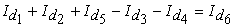(2)

Using drain current equation of all the MOSFETs operating in triode region in terms of Vgs and Vds, from (2), the voltage output of the circuit is found to be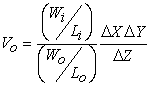(3)

where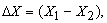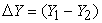and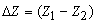. Thus, like the circuits of [5, 6], the proposed circuit can perform the computation of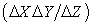where all the signals are differential input voltages and if aspect ratios of all MOS transistors are taken to be same, the output voltage becomes independent of the parameters of the MOS transistors.

In order to keep operation of all the MOS transistors in the linear region one requires:

For M1 to M4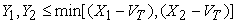(4)

For M5 to M6: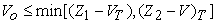(5)

where VT is the threshold voltage of the MOS transistors.

### 3. SPICE Simulation Results

To verify the workability of the proposed configuration, a number of its applications have been checked through SPICE simulations using the CMOS CDBA of  biased with +5V DC supply while using MOS transistors with 0.35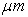CMOS process parameters.

3.1. Analog Divider

For checking the divider operation, input signals were applied at Y and Z terminals and the control voltages were applied at X terminal of the MOSFETs. Taking Y1 = Y2 = y, X1 = Vc1 and X2 = Vc2, Z1 = (Z + z) and Z2 = (Z –z), where Z is the DC bias at gate terminal of M5 to M6 and Vc1, Vc2 are DC control voltages, we obtain output voltage as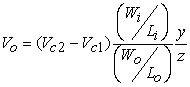(6)

The conditions required to keep operation of the MOS transistors in the linear region are given by

For M1 to M4: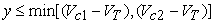(7)

For M5 to M6: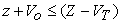(8)

In SPICE simulations of the divider circuit, Vc1 and Vc2 were kept at 5.0V and 4.95V respectively while Z was kept at 4.5V. The input signal z was varied from -2V to -0.6V and y signal was varied from -0.2V to 0.2V in steps of 0.1V. With all MOSFETs having same aspect ratios, the simulation results and theoretical results are shown in Figure 3 which are found to be closely matching with the theoretical ones.

3.2. Analog Multiplier

In this case, the input signals are applied at the X and Y terminals. Let Y1 = -Y2 = -y, X1 = (X+x) and X2 = (X-x), Z1 = Vc1 and Z2 = Vc2, where X is the DC bias at gate terminal of M1 to M4 and Vc1, Vc2 are DC control voltages. Using these signals and control voltage, we obtain the output voltage of the circuit as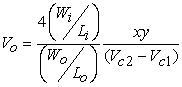(9)

The condition required to keep operation of the MOS transistors in the linear region is given by

For M1 to M4: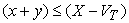(10)

The widths and lengths of M1 to M6 were taken as 2.8 µm and 0.35 µm respectively; X was kept at 4.0V while Vz1 and Vz2 were kept at 3.75V and 5V respectively. The x input signal was varied from –0.23V to 0.23V and y signal was varied from -180mV to 180mV in step of 90mV. Figure 4 shows the simulation results which are seen to be in good agreement with the theoretical ones.

3.3. Squarer

For using the circuit as a squarer, X was kept at 4.5V while Vz1 and Vz2 were kept at 3.75V and 5V respectively. The inputs x and y were both taken as sinusoidal signals of 1 KHz, zero avg., 200mVpp. Figure 5 shows the simulation results of the proposed configuration as a squarer.

3.4. Modulator

To verify the operation of the proposed configuration as a modulator, X was kept at 4.5V while Vz1 and Vz2 were kept at 3.75V and 5V respectively. The input x was sinusoidal signal of 1 KHz, zero avg., 200mVpp while y was taken as a sinusoidal signal of 10 KHz, zero avg., 200mVpp. Figure 6 shows the simulation results of the circuit as a modulator.

The SPICE simulation results of Figure 3-Figure 6, thus, establish the workability of the proposed configuration in its various modes of operation.

3.5. Frequency Characteristics of the Proposed Configuration

In this case, the input X was kept at 4V while Vz1 and Vz2 were kept at 3.75V and 5V respectively. The input x was taken as a sinusoidal signal of amplitude 1mV of varying frequency and y was taken as a DC signal of 0.1V. Figure 7 shows the frequency response of the proposed configuration obtained through SPICE simulations which is seen to exhibit a bandwidth of around 82.3MHz.

Figure 7. Frequency response of the proposed Multiplier/Divider cell obtained through SPICE simulations

### 4. Concluding Remarks

A new multiplier/divider cell based on a single CDBA is presented. While previously known single-CDBA-four-MOSFETs-based circuits can realize only a multiplier as in  or only a divider as in  (with the two CFOAs employed therein configured exactly equivalent to a CDBA as mentioned in footnote 1), the presented single-CDBA-based configuration uses six MOSFETs but has the advantage of realizing both multiplier and divider functions simultaneously. SPICE simulation results based on CMOS CDBA and MOS transistors in 0.35um CMOS technology have confirmed the workability of the proposed configuration in its various modes of operation. The layout of the proposed cell using CMOS CDBA architecture from  has shown the chip area of the circuit as 0.015mm2 (layout area without pads).

### Acknowledgement

This work was performed at Analog Signal Processing Research Lab and Circuit Simulation Lab of NSIT, New Delhi. Thanks are due to Ankur Sangal of Coreel Technology India Pvt. Ltd. for his assistance with the layout design.

### References

  Acar, C. and Ozoguz, S, “A new versatile building block: Current differencing buffered amplifier suitable for analog signal processing filters,” Microelectronics journal, 30. 157-160.1999.In article  Acar, C. and Sedef, H, “Realization of nth order current transfer function using current differencing buffered amplifiers,” International of Electronics, 90(4). 277-283. 2003.In article CrossRef  Tangsrirat, W., Klahan, K., Dunmawipata, T. and Surakampontorn, W., “Low-voltage NMOS-based current differencing buffered amplifier and its application to current-mode ladder filter design,” International Journal of Electronics, 93(11). 777-791.2006.In article  D. Biolek, D., Bajer, J., Biolkova, V., Kolka, Z. and Kubrick, M, “Z-copy-controlled Gain-current differencing buffered amplifier and its applications,” International Journal of Circuit Theory and Applications, 39.257-274. 2010.In article  Abuelma’atti, M.T. and Al- Qahtani, M.A, “A current- mode current-controlled current-conveyor based analogue multiplier/ divider,” International Journal of Electronics, 85(1). 71-77. 1998.In article CrossRef  Premont, C., Abouchi, N., Grisel, R. and Chante, J, “A BiCMOS current conveyor based four-quadrant analog multiplier,” Analog integrated circuits and signal processing, 19. 159-162. 1999.In article CrossRef  Yuce, E, “Design of simple current-mode multiplier topology using a single CCCII+,” IEEE Transaction on Instrumentation and Measurement, 57(3). 631-637. 2008.In article CrossRef  Kaewdang, K., Fongsamut, C. and Surakampontorn, W, “A wide band current-mode OTA based. Analog multiplier-divider,” Proceedings of the 2003 International Symposium on Circuits and Systems, 1.349-352. 2003.In article CrossRef  Tangsrirat, W., Pukkalanun, T., Mongkolwai, P. and Surakampontorn, W, “Simple current-mode analog multiplier, divider, square-rooter and squarer based on CDTAs,” International Journal of Electronics, 65 (3). 198-203. 2011.In article CrossRef  Khachab, N. I. and Ismail, M, “MOS multiplier/divider cell for analogue VLSI,” Electronics Letters, 25(23), 1550-1551. 1989.In article CrossRef  Khachab, N. I, “A nonlinear CMOS analog cell for VLSI signal and information processing,” IEEE Journal of Solid State Circuits, 26(11). 1689-1699. 1991.In article CrossRef  Ismail, M., Brannen, R., Takagi, S., Fujii, N., Khachab, N. I., Khan, R. and Asserud, O, “Configurable CMOS multiplier/divider circuits for analog VLSI,” Analog Integrated Circuits and Signal Processing, 5.219-234. 1994.In article  Pandey, R., Pandey, N., Sriram, B. and Paul, S. K, “Single OTRA based analog multiplier and its applications,” International Scholarly Research Network,” 2012, Article ID 890615, 7, 2012.In article  Liu, S. I., and Chen, J. J, “Realization of analogue divider using current feedback amplifier,” IEE Proc. Circuits Devices System, 142(1). 45-48. 1995.In article  Keskin, A. U, “A Four quadrant analog multiplier employing single CDBA,” Analog Integrated Circuits and Signal processing, 40. 99-101. 2004.In article CrossRef  Siripruchyanun, M, “A design of analog multiplier and divider using current controlled current differencing buffered amplifiers,” Integrated Circuits, 2007. ISIC '07. International Symposium on Components, Circuits, Devices & Systems, Engineered Materials, Dielectrics and Plasmas, 568-571. Sept. 2007.In article  Maheshwari, S. and Khan, I. A, “Current controlled current differencing buffered amplifier: implementation and applications,” Active and Passive Electronic components, 4. 219-227. Dec. 2004.In article CrossRef  Jaikla, W. and Siripruchyanun, M, “Novel current mode four quadrant multiplier/divider based on current controlled CDBAs (CCCDBAs),” The 2006 ECTI International Conference, 509-512. 2006.In article  Riewruja, V. and Rerkratn, A, “Four-quadrant analogue multiplier using operational amplifier,” International Journal of Electronics, 98(4). 459-474. 2011.In article CrossRef  Woratraijariya, P., Mano, K., Jaikla, W. and Maneewan, S, “Current-mode 4 quadrant divider employing only single active element,” Electron Devices and Solid State Circuit (EDSSC), 2012 IEEE International Conference, 1-4. 2012.In article  Kumngern, M, “A DXCCII-based Four-quadrant multiplier,” Power Engineering and Optimization Conference (PEOCO), 2013 IEEE 7th International Conference, 738-741. 2013.In article  Siripruchyanun, M. and Jaikla, W, “A current-mode analog multiplier/divider based on CCCDTA,” AEU - International Journal of Electronics and Communications, 62(3). 223-227. 2008.In article CrossRef

### Notes

1A closer look at the circuit of Fig.3 of  reveals that the composite connection of the two CFOAs therein is exactly configured as a CDBA! Hence, the two-CFOA-based analog divider of Fig.3 of  can also be considered to be single-CDBA-four-MOSFETs-based analog divider.